# Subsampled Circulant Matrix Based Wideband Spectrum Sensing Using Fusion Based Recovery Algorithm

Subsampled Circulant Matrix Based Wideband Spectrum Sensing Using Fusion Based Recovery Algorithm

Corresponding Author Email:
aswini.thota@srivasaviengg.ac.in
Page:
1201-1208
|
DOI:
https://doi.org/10.18280/ts.380431
9 April 2020
|
Accepted:
25 July 2021
|
Published:
31 August 2021
| Citation

OPEN ACCESS

Abstract:

This paper reflects the problem of wideband spectrum recovery. The demand for spectrum usage is increasing exponentially as the wireless technologies rules the world. To meet these needs, Cognitive Radio is one of the emerging technologies, which intelligently allots the spectrum to the secondary users. Since the spectrum is wideband, the capability of spectrum sensing is improved by introducing sub-nyquist sampling under compressive sensing framework. In this paper, a sub-nyquist sampling technique of Modulated Wideband Converter (MWC) is used as it possesses m-parallel channels providing fast sensing and robust structure. A circulant matrix method is used to improve the hardware complexity of MWC. Also at the reconstruction of MWC, a fusion based recovery algorithm is proposed which became an added benefit for perfect recovery of the support. The results are compared with conventional MWC in terms of support recovery, mean square error and SNR gain. Simulations proved that the proposed algorithm performs superior at low as well as high SNR with increased gain.

Keywords:

modulated wideband converter, circulant matrix, deterministic sequence, compressive sensing, orthogonal matching pursuit

1. Introduction

Now-a-days, the digital world rules our everyday life. Telecommunications, medical services, education and entertainment need digital media. The existence of digital world is possible with perfect analog to digital conversion. This development has increased the demands of wideband spectrum and paved way to a new radio frequency (RF) technology of cognitive radio. This has led to spectrum congestion. Cognitive Radio (CR) is an intelligent software defined radio which intelligently allots the unused spectrum to the secondary users. Of all the different works of CR, spectrum sensing plays a vital role. A major challenge is to sample the wideband signal at high rates satisfying good resolution. The well-known Shannon-Nyquist sampling theorem says that a signal which is bandlimited can be sampled and reconstructed perfectly provided the sampling rate must exceed twice its bandwidth. The signal here is a wideband signal which cannot be processed by normal analog-to-digital converters (ADC). Due to its low spectrum utilization, the wideband spectrum is inherently sparse, hence applying the concept of compressive sensing (CS), samples the wideband signals at sub-Nyquist rates [1-4]. Under CS framework, most of the proposed architectures included random demodulator (RD) for spectrally sparse signals, periodic non-uniform sampling (PNS) for sparse multitone signals  and modulated wideband converter (MWC)  for sparse multiband signals.

The Modulated Wideband Converter (MWC) is a multichannel architecture which mixes a multiband signal with a periodic random sequence and passed through a low rate sampler. It is constructed with m channels with parallel structure in which each channel comprises of a mixing function, a low pass filter (LPF) and an analogue-to-digital converter (ADC). Due to its flexible design and parallel structure, it is used in many practical wireless applications like cognitive radio, channel estimation, radar, etc., This paper focuses on two problems and improves the performance of MWC. The first problem focuses on hardware complexity of MWC [7, 8] in which the measurement matrix of length m´M plays a vital role. The random sequence of Bernoulli or Gaussian is considered with a length of M. Now m´M measurement matrix is constructed by circular shifting the sequence m times or taking m different combinations of the sequence. In this process, it needs mM flip-flops of shift registers. Some binary sequences like deterministic sequences which are identical to random Bernoulli matrices, also suitable for this case. The deterministic sequences are not fully random. Hence to reduce the hardware complexity of MWC, alternating methods of using deterministic sequences is preferred which guarantees better reconstruction and good choice of hardware. Out of all the other sequences, the maximum length sequence, Gold, Kasami codes exist for a finite prime length of 2n-1 where n is the degree of the primitive polynomial and nÎM, which are not feasible for all applications [8-13]. The Legendre sequence is one of the deterministic sequence exist for any choice of length provided it should be a prime. Li et al.  proposed a circulant matrix structure in which each row is attained by random cyclic shift of the deterministic sequence. This circulant measurement matrix provides memory efficient hardware since only M flip-flops are required and only M log M multiplications are needed indicating it is also faster [14-17].

The second problem is to find the suitable reconstruction algorithm [18, 19] which improves the support recovery performance of MWC. In this regard, a fusion based algorithm [20-24] is proposed and compared with existing simultaneous orthogonal matching pursuit (Somp) algorithm.

Organization of the paper is as follows: section II gives the related background of compressed sensing. A sparse multiband model and sub-nyquist sampling scheme are outlined in Section III. Section IV describes the proposed reconstruction algorithm. Numerical and simulation results are given in section V.

In this paper, matrices are denoted with upper case as X and vectors with lower case letters as x. $X \in \mathbb{R}^{m \times N}$ is an mxM matrix with all entries corresponds to real values. T or * represents transpose of a matrix. Also $\dagger$ is a pseudo inverse form of a matrix A which means $A^{\dagger}=\left(A A^{T}\right)^{T}$. p-norm calculations are indicated by ||.||p.

2. Related Background of Compressed Sensing

The Compressive Sensing (CS) is a new framework of sampling the signals which are sparse at any basis. Consider a real vector of finite length, discrete time signal $X \in \mathbb{R}^{M}$. To make it sparse, it is represented using an arbitrary basis $\left\{\psi_{i}\right\}_{i=1}^{M}$, a set of orthonormal vector in the space $\mathbb{R}^{M}$ . An orthonormal matrix is formed by these vectors as $\Psi \in \mathbb{R}^{M \times M}$. Now the signal is given as

$\mathbf{x}=\sum_{i=1}^{M} \mathbf{z}_{i} \boldsymbol{\psi}_{i}$ or $\mathbf{x}=\Psi \mathbf{z}$                      (1)

where, z is the projection of x over Ψ. Thus the signal x in time domain is equal to z in Ψ domain. The signal x is measured by sampling it with a measurement matrix $A \in \mathbb{R}^{m \times M}$. The measurements y at each row corresponds to the inner product $y=\langle\phi, x\rangle$ where, ϕ belongs to the rows of A . Thus the measurements are given as

$\mathbf{y}=\mathbf{A} \mathbf{x}=\mathbf{A} \Psi \mathbf{z}$                      (2)

If mM, then x is perfectly reconstructed from its measurements y. If m≤M, it becomes an ill-posed problem which has infinite number of solutions.

Consider z is a K-sparse signal under the basis of Ψ where $K \ll M$. Then $K \ll m \ll M$ is an underdetermined solution. To solve this, the measurement matrix must obey RIP (Restricted Isometry Property) such that $m \geq C \cdot K \log \left(\frac{M}{K}\right)$ where C is constant. Also the incoherence between measurement and basis matrices provides better stability for measurement matrix. Clearly the solution for this inverse problem is to find the Least square solution Eq. (2) i.e., $\hat{x}=\left(A A^{T}\right)^{-1} A^{T} y, \quad \ell_{p}-\text{norm}$ calculations provide the sparsest solution.

Some convex optimization techniques like basis pursuit algorithms are accurate but consume high recovery time. Also the recovery is possible by many greedy algorithms which are iterative [20-22]. Mostly, it includes orthogonal matching pursuit (omp) and its derivatives provide faster recovery.

3. Signal Model and Sub-Nyquist Sampling Scheme

Consider an analog multiband signal x(t) whose fourier transform X(f) is bandlimited to $-\frac{f_{N Y Q}}{2} \mathrm{to} \frac{f_{N Y Q}}{2}$ Hz. The total wideband communication model is divided into subbands each of BHz bandwidth. It consists of N active bands centered at frequency fn, n=1,2,…,N. Thus x(t) is a sparse wideband signal with $N B \ll f_{N Y Q} .$. The positions of the bands are random with no prior information. Figure 1 depicts the assumption of wideband spectrum. In CR context, these active bands are termed as occupied bands of primary users and the remaining subbands of the model are unoccupied bands called spectrum holes. The main idea of CR is to sense these spectrum holes and allot to secondary users.

## 1.png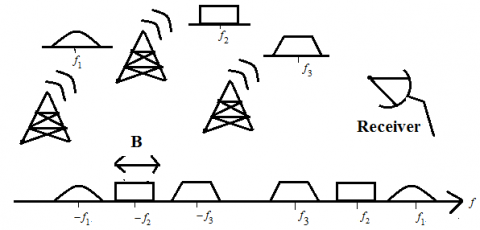Figure 1. Wideband spectrum with three different frequencies of multiband signal

3.1 MWC sampling method

Modulated wideband converter is one of the sub-nyquist sampling methods which consist of m-parallel channels . The multiband signal x(t) is applied to m channels simultaneously. At ith channel, the signal x(t) is mixed with a periodic random sequence pi(t) which alternates between +1 and -1.

$p_{i}(t)=\sum_{l=-\infty}^{\infty} a_{i l} e^{j 2 \pi l t / T_{p}}$                        (3)

where, Tp is the sampling period.

This mixing spreads the signal over the period Tp represented in time domain as $\tilde{x}_{i}(t)=x(t) \cdot p_{i}(t)$ ie., in fourier domain $\tilde{x}_{i}(t)$ is fp shifted replicas of X(f). The entire architecture of MWC is depicted in Figure 2.

## 2.png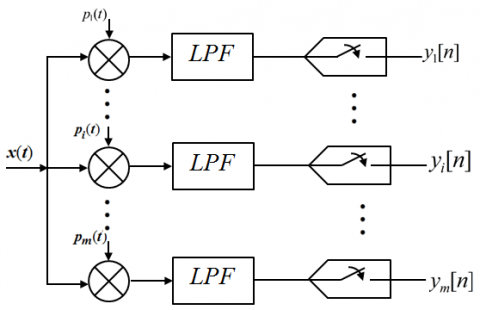Figure 2. Architecture of modulated wideband converter

Then the mixed signal is passed through a LPF truncating with cutoff frequency of $\frac{1}{2 T_{S}}$. The low pass filtered signal is now sampled at t=nTs to get the sampled measurements. Thus the DTFT of the measurements yi[n] is expressed as

$Y_{i}\left(e^{j 2 \pi f T_{S}}\right)=\sum_{l=-L_{0}}^{L_{0}} a_{i l} X\left(f-l f_{p}\right)$                      (4)

where, $L_{0}=\left(f_{N Y Q}+\frac{f_{s}}{2 f_{p}}-1\right)$.

The sign alternating function pi(t) is generated by a random sequence alternated between +1 and -1 for M equal time intervals. This is given as

$p_{i}(t)=\alpha_{i k}, \frac{k T_{p}}{M} \leq t \leq(k+1) \frac{T_{p}}{M}$                     (5)

where, $\alpha_{i k} \in[-1,+1] .$ It is an $m \times M$ pseudo random matrix referred as S. Now the measurements expressed in Eq. (4) is modified and represented in matrix form as

$y(f)=S F D Z(f)=S_{m \times M} F_{M \times L} D_{L \times L} Z(f)$                       (6)

where, S is sign matrix, F is DFT matrix and D is diagonal matrix with dl defined as

$d_{l}=\frac{1}{T_{p}} \int_{0}^{T_{p} / M} e^{-j 2 \pi l t} / T_{p} d t=\begin{array}{cc}\frac{1}{M} & l=0 \\ \frac{1-\theta}{2 j \pi l} & l \neq 0\end{array}$                       (7)

Since Eq. (6) is the key to recover the signal, finally the measurements are obtained as y(f)=AZ(f) in which A is the measurement matrix.

3.2 Circulant matrix design

The sign matrix SmxM is implemented by shift registers in which the number of filps-flops used is justified by M. To reduce the hardware complexity of MWC, alternating methods of using deterministic sequences is preferred which guarantees better reconstruction and good choice of hardware. Out of all the other sequences, the maximum length sequence, Gold and Kasami codes exist for a finite prime length of 2n-1 where n is the degree of primitive polynomial and n$\in$M, which are not feasible for all applications. The Legendre sequence is one of the deterministic sequence exist for any choice of length provided it should be a prime . A Legendre sequence is defined as

$L S_{i}=\left\{\begin{array}{c}L S_{0}=1 \\ 1, i \text { is square } \\ -1, i \text { is non-square }\end{array}\right.$                     (8)

Theorem-1: Consider an mxM circulant matrix $A=\frac{1}{\sqrt{m}} R U$, where $\frac{1}{\sqrt{m}}$ is a normalizing factor, R is a random sampling operator which randomly selects m out of M ones uniformly, U is an MxM unitary matrix constructed by a deterministic sequence which satisfies $\mathrm{U}^{*} \mathrm{U}=\mathrm{MI}_{M}$, provided the deterministic sequence should have a flat spectra.

The mixing sequence S is constructed by a circulant matrix design proposed  according to Theorem 1 represented as

$\mathrm{S}=\frac{1}{\sqrt{m}} \mathrm{RC}$                      (9)

where, C is an MxM circulant matrix and R is a random operator which randomly selects m rows of matrix C.

The circulant matrix C is constructed from any deterministic sequence. Let the sequence be a Legendre sequence with a length of M considered as $c=\left[L S_{0}, L S_{1}, L S_{2}, \ldots \ldots . L S_{M-1}\right]$. This is taken as the first row of circulant matrix and next rows are obtained by cyclic shifting c right/left to generate an MxM matrix which is given as

\begin{aligned} C &=\left[\begin{array}{cccc}L S_{0} & L S_{1} & \cdots & L S_{M-1} \\ L S_{M-1} & L S_{0} & \cdots & L S_{M-2} \\ \vdots & \vdots & \ddots & \vdots \\ L S_{1} & L S_{2} & \cdots & L S_{0}\end{array}\right] \\ &=\mathrm{F}^{-1}\left[\begin{array}{cccc}L S_{0} & 0 & \cdots & 0 \\ 0 & L S_{1} & \cdots & 0 \\ \vdots & \vdots & \ddots & \vdots \\ 0 & 0 & \cdots & L S_{M-1}\end{array}\right] \mathrm{F} \end{aligned}                         (10)

So, finally the measurement matrix is given as

$\mathbf{S}=\frac{1}{\sqrt{m}} \mathbf{R}\left[\begin{array}{cccc}L S_{0} & L S_{1} & \cdots & L S_{M-1} \\ L S_{M-1} & L S_{0} & \cdots & L S_{M-2} \\ \vdots & \vdots & \ddots & \vdots \\ L S_{1} & L S_{2} & \cdots & L S_{0}\end{array}\right]$                            (11)

where, S is measurement matrix, $\frac{1}{\sqrt{m}}$ is the normalizing factor and R is random sampling operator which randomly selects m out of M ones Thus the deterministic sequence (here Legendre sequence) is cyclic shifted M times to form a circulant matrix. Then mixing sequence S is formed by random selection of m rows to get m´M matrix. A mixing function with random Bernoulli matrix use mM flip-flops, however with the use of deterministic sequence such as Legendre requires only M flip-flops reducing the hardware complexity.

4. Reconstruction

4.1 CTF block

After receiving the measurements y[n], Continuous-to-finite (CTF) block plays an important role in the recovery of support vector as shown in Figure 3. In y(f)=AZ(f), A is m´L matrix in which m<L shows an underdetermined equation . Hence it can be solved by building multiple measurement vector (MMV) system.

## 3.pngFigure 3. Continuous-to-finite (CTF) block

The difficulty of infinite measurement vector problem makes the direct reconstruction of signal $\hat{x}(t)$ is not possible. This can be solved by using Continuous-to-finite (CTF) method. Hence, first frame v is constructed which satisfies the relation $Q=v v^{H}$ where Q is the measurement set defined as

$\mathrm{Q}=\sum_{n=-\infty}^{\infty} y[n] y^{T}[n]$                    (12)

Now solving for MMV system v=Au by applying any greedy algorithms attains the support set. Once the support is found, reconstruction can be done by

$\mathbf{z}_{s}(f)=\mathbf{A}_{s}^{\dagger} \mathbf{y}(f)$                    (13)

where, zs(f) is DFT of $\mathrm{Z}[n], A_{s}^{\dagger}$ is the pseudo-inverse of A indexed by support columns and y(f) is the measurement vector. zi[n] is now interpolated to Nyquist rate and these sequences are finally modulated in time domain to get $\hat{x}(t)$.

In this context, to reconstruct the signal $\hat{x}(t)$, the reconstruction algorithm plays a major role. Among the CS reconstruction algorithms, some convex optimization techniques like basis pursuit algorithms are accurate but consume high recovery time. Also the recovery is possible by many greedy algorithms which are iterative. Mostly, it includes Orthogonal Matching Pursuit (OMP) and its derivative algorithms provide faster recovery but reduced performance than convex. In this paper, a fusion based recovery algorithm is proposed by combining both convex and greedy algorithms to achieve higher accuracy in recovery of the signal. In this context, Simultaneous orthogonal matching pursuit (Somp) and Subspace Pursuit (SP) algorithms are combined.

4.2 Extended SP embedded SOMP algorithm

Of all the recovery algorithms of CS, greedy algorithms  are in general harder to analyze but computationally efficient, easy to implement and also provide better performance. Orthogonal Matching Pursuit (OMP) is the most popular due to its better reconstruction performance and simple structure. Out of the many derivative algorithms of OMP, Simultaneous orthogonal matching pursuit (Somp)  has drawn its interest for the recovery support of MWC. The support set is explained in the following definition.

Definition: The support set Ʌ is a set of indices corresponding to the non-zero or active elements of the sparse vector x:

$\Lambda \triangleq\left\{i: x_{i} \neq 0\right\}$

and its complement is $\bar{\Lambda} \triangleq\left\{i: x_{i}=0\right\}$ then $\Lambda \cup \bar{\Lambda}=\{1,2, \ldots \ldots N\}$ and $\Lambda \cap \bar{\Lambda}=\phi$              (14)

In Somp algorithm, at kth iteration the index corresponding to the highest magnitude of correlation between matrix A and residual rk-1 is identified. Then it is added to the support set Ʌ along with the symmetric location of the index. Later the sub matrix As is obtained as the matrix A indexed by support set columns. Now using least square method the approximate solution is found to update the residual rk. Thus Somp, variant of OMP, has proved its better reconstruction performance.

The main limitation of Somp is lack of self-correcting procedure while estimating the support set. Conversely, Subspace Pursuit (SP) itself possesses self-correction method . Thus Algorithm 1is a different method proposed to incorporate a self-correcting mechanism using SP in Somp. The step 4 checks the estimated support using SP algorithm.

 Algorithm 1: SP embedded Somp Input An mxM circulant matrix A mx2N frame vector v Support recovery N Initialization k=0, $\Lambda^{\text {Somp }}=\varnothing$ null vector, residual r=V Procedure k=k+1 $\lambda_{k}=\underset{j=1,2, \ldots L}{\operatorname{argmax}} \frac{\left|\left\langle A_{j} r_{k-1}\right\rangle\right|}{d}$ where d is the norm of diagonal elements of A $\Lambda^{\text {Somp }}=\lambda_{k} \cup \lambda_{k s y m m} \cup \Lambda_{k-1}$ $\left[\Lambda_{k}^{(S P)}\right]=S P\left(\mathbf{A}, \mathbf{v}, i, \Lambda^{(\text {Somp })}\right)$ $\Lambda_{k}=\Lambda_{k}^{(S P)}$ $\mathbf{r}_{k}=\mathbf{v}-\mathbf{A}_{\Lambda_{k}} \mathbf{A}_{\Lambda_{k}}^{\dagger} \mathbf{v}$ Repeat until (k≤N) Output: Support set Ʌ

The Algorithm2 describes the Subspace Pursuit in which Ʌinit is the previous support set. By taking N1 highest magnitude elements of correlated vectors A and rk, it obtains the least square solution approximation. At kth iteration, estimated support set is found by adding or deleting indices. Thus it gives the correct support set which improves the performance of Somp.

 Algorithm 2: Subspace Pursuit Initialization Input An mxM circulant matrix A mx2N frame vector v Support recovery N1 Initialization: $k=0, \Lambda_{0}=\Lambda_{\text {init }}$, residual $\mathbf{r}=\mathbf{v}-\mathbf{A}_{\Lambda_{0}} \mathbf{A}_{\Lambda_{0}}^{\dagger}$ Ensure    $\left|\Lambda_{\text {init }}\right| \leq N_{1}$ Procedure k=k+1 j indices of N1 maximum components of $\lambda=\underset{j=1,2, \ldots L}{\arg \max }\left|\left\langle\mathbf{A}_{j} \mathbf{r}_{k-1}\right\rangle\right| / d$ where d is the norm of diagonal elements of A. $\Lambda=\lambda \cup \Lambda_{k-1}$ $\tilde{\mathbf{x}}_{\Lambda}=\mathbf{A}_{\Lambda}^{\dagger} \mathbf{v}$ $\Lambda_{k}=$ indices of N1 largest magnitude of $\tilde{\mathbf{x}}_{\Lambda}$ $\mathbf{r}_{k}=\mathbf{v}-\mathbf{A}_{\Lambda_{k}} \mathbf{A}_{\Lambda_{k}}^{\dagger} \mathbf{v}$ Repeat until ($\left\|\mathbf{r}_{k}\right\|_{2} \geq\left\|\mathbf{r}_{k-1}\right\|_{2}$) Output $\Lambda=\Lambda_{k-1}$

As described by Algorithm1, SP embedded Somp is same as Somp in estimating the support set. After finding the support Ʌk in the kth iteration, it is refined using SP algorithm as in step 4. This self-correcting process of SP used in Somp is renamed as SP embedded Somp.

Algorithm 1 is run for kN iterations to estimate the support. To identify the exact non-zero elements, Sahoo and Makur  proposed an idea to extend the number of iterations.

Theorem 2: Suppose that x is an arbitrary k-sparse multiband signal with a measurement matrix Φ of size mxM can be recovered, by fixing $\alpha \in[0,1]$,, in at most k+[αk] iterations.

Algorithm 3 describes that algorithm 1 is proposed to run at k+[αk] iterations according to Theorem 2. Thus Extended SP embedded Somp (ESPsomp) selects more atoms. This increases the additional computational cost by a factor of 1+α which can be discarded with an increase of recovery performance.

 Algorithm 3: Extended SP embedded Somp (ESPsomp) This is same as algorithm 1 except the following change Until k+[αk] where $\alpha \in[0,1]$
5. Simulation Results

The simulations are done using MATLAB R2015 with Intel (R) Core (TM) i5-4790 CPU @ 3.60 GHz and 8 GB RAM on a PC. The system is a 64-bit Microsoft Windows-7 operating system. Table 1 lists the parameters used to construct a wideband signal x(t).

$x(t)=\sum_{i=1}^{3} \sqrt{E_{i} B} \sin c\left(\beta\left(t-\tau_{i}\right)\right) \cos \left(2 \pi f_{i}\left(t-\tau_{i}\right)\right)$                       (15)

Table 1. Parameters used for wideband signal

 Parameter Value fNYQ 10 GHz B 50 MHz Time offset τi {0.4,0.7,0.2} μs Energy Ei {1,2,3} fs=fp 50.76 MHz No. of channels (m) 50 M=L 197

According to the signal model, an analog multiband signal x(t) is considered with N= 3 active bands (support) centered at three different frequencies. In the simulations, M=197, the prime number is chosen to generate the Legendre sequence. 200 Monte–Carlo simulations were done in this process. The performance of the system using circulant measurement matrix design is compared with different sequences like Legendre, Bernoulli and Zadoff-chu sequences. Figure 4a and 4b depicts the support recovery at different SNR ranging from -10 to 25 dB using ESPomp and somp reconstruction algorithms respectively. The proposed recovery algorithm outperforms Somp in terms of percentage of support recovery. The results of using ESPomp and Somp for different sequences are also tabulated in Table 2 and Table 3 respectively. This shows that the construction of circulant matrix using Legendre sequence proved its performance in terms of success rate (support recovery) as well as hardware complexity.

Table 2. Comparison of percentage of support recovery for different sequences using ESPsomp algorithm

 Legendre Bernoulli Zadoff-chu SNR = 5 dB 69.5% 52.5% 18% SNR = 10 dB 95% 91% 39% SNR = 15 dB 100% 98.5% 59%

Table 3. Comparison of percentage of support recovery for different sequences using Somp algorithm

 Legendre Bernoulli Zadoff-chu SNR = 5 dB 68% 46.5% 19.5% SNR = 10 dB 93% 87% 39% SNR = 15 dB 99% 97.5% 58%

## 4.png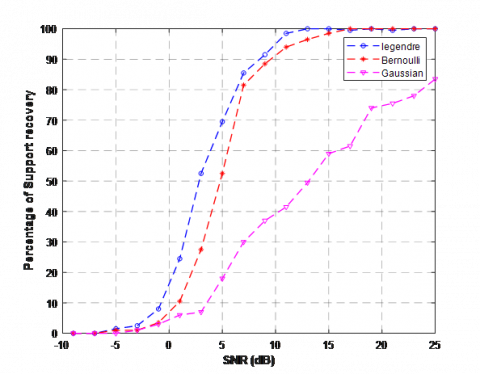Figure 4a. Percentage of support recovery vs SNR for different sequences using ESPsomp algorithm

## 4b.png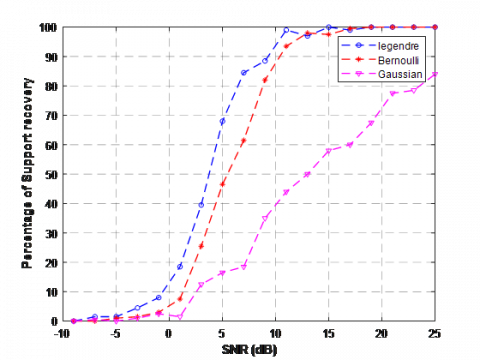Figure 4b. Percentage of support recovery vs SNR for different sequences using Somp algorithm

## 5.png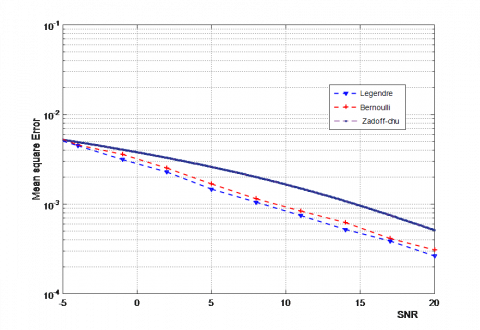Figure 5. MSE performance for different sequences

## 6.png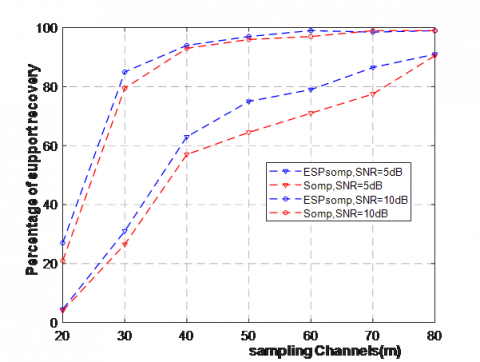Figure 6. Support recovery at increasing channels for proposed algorithm

## 7.png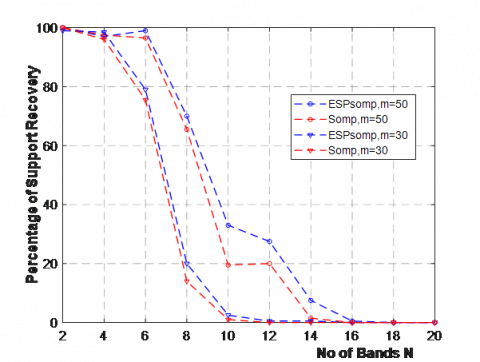Figure 7. Percentage of support recovery at varying frequency bands

Considering the performance of the proposed recovery algorithm for different sequences at various metrics. Mean Square Error (MSE) is one of the performance metrics calculated as

$M S E=\frac{\|\hat{x}-x\|_{2}}{\|x\|_{2}}$                        (16)

The averaged MSE at varying SNR of -15 to 25 dB was compared for different deterministic sequences using proposed algorithm. Lesser the value of MSE, better the performance of the design. It is clearly shown in the Figure 5 that the Legendre sequence acquired better MSE compared with well-known Bernoulli and Zadoff-chu sequences. The averaged MSE performance of the sequences using ESPsomp is given in Table 4. At SNR=10dB, MSE of Zad0ff-chu has 21.38´10-4 whereas Legendre is with only 9.79´10-4.

Table 4. Comparison of MSE for different sequences

 Legendre Bernoulli Zadoff-chu SNR = 5 dB 5.49´10-3 1.8´10-4 5.08´10-3 SNR = 10 dB 9.79´10-4 10.45´10-4 21.38´10-4 SNR = 15 dB 1.8´10-4 1.97´10-4 18.76´10-4

Also the comparison is done in terms of output SNR and SNR gain which are tabulated in Table 5 and Table 6. Output SNR of the design is calculated as

Output $S N R=20 \log \|x\|_{2} /\|x-\hat{x}\|_{2}$                      (17)

The output SNR indirectly acts as a metric for sensing gain. And Sensing gain is the difference of input SNR to output SNR. Higher the sensing gain, better the recovery performance. At input SNR of 15 dB, the Legendre sequence outperforms with output SNR of 19.38 dB achieving a gain of 4.38 dB, however output SNR is only 15.22dB with only 0.22 dB gain for Zadoff-chu.

Table 5. Comparison of output SNR for different sequences

 Legendre Bernoulli Zadoff-chu SNR = -15 dB -10.67 dB -11.53 dB -8.81 dB SNR = 5 dB 9.35 dB 8.37 dB 7.4 dB SNR = 15 dB 19.38 dB 18.51 dB 15.22 dB

Table 6. Comparison of Sensing gain for different sequences

 Legendre Bernoulli Zadoff-chu SNR = -15 dB 4.32 dB 3.46 dB 6.18 dB SNR = 5 dB 4.35 dB 3.37 dB 2.4 dB SNR = 15 dB 4.38 dB 3.35 dB 0.22 dB

Now the impact of circulant matrix using Legendre sequence on the system is compared using proposed recovery algorithm. The simulations are done at N=6 and SNR 5 dB & 10 dB at varying channels from 20 to 80. As shown in Figure 6, the success recovery is always high using ESPsomp than Somp. Also Figure 7 shows the success recovery of proposed algorithm at varying sparcity (bands) from 2 to 20. The parameters of SNR=10 dB, channels m=30 & 50 at random energy levels are taken following the symmetric frequency bands. As the number of bands increasing, the recovery performance decreases. This is because, as the sparse bands increases, the sparsity of the original signal rises demanding more number of channels. As N≥14, the support recovery is very less for Somp. However ESPsomp performs better than Somp.

6. Conclusions

In this paper, a sub-Nyquist sampling scheme of MWC is used as a mechanism to meet the needs of spectrum congestion. To avoid the hardware complexity of MWC, a circulant measurement matrix is proposed with deterministic sequences. Among the different deterministic sequences, Legendre sequence outperforms by achieving better gain and MSE. Traditionally, the measurement matrix with Bernoulli use mM flip-flops, however with the use of deterministic sequence such as Legendre requires only M flip-flops reducing the hardware complexity. Also at the reconstruction of MWC, the proposed fusion based recovery algorithm becomes an added benefit for perfect recovery of the support. Simulations proved that the proposed Extended SP embedded Somp (ESPsomp) algorithm performs superior at low as well as high SNR with increased gain. However, the increased additional computational cost by a factor of 1+α can be discarded with an increase of recovery performance.

Nomenclature
 A measurement matrix X signal vector Z projection vector y measurement vector N number of active bands LS legendre sequence m number of channels M length of the sequence $\tilde{\mathbf{x}}$ recovered vector S measurement matrix Greek symbols $\Psi$ basis vector $\Lambda$ support vector $\lambda$ observation vector $\alpha$ run factor $\tau$ time offset Subscripts k iterations NYQ nyquist frequency
References

 Donoho, D.L. (2006). Compressed sensing. IEEE Transactions on Information Theory, 52(4): 1289-1306. https://doi.org/10.1109/TIT.2006.871582

 Baraniuk, R.G. (2007). Compressive sensing. IEEE Signal Processing Magazine, 24(4): 118-121. https://doi.org/10.1109/MSP.2007.4286571

 Candès, E.J., Wakin, M.B. (2008). An introduction to compressive sampling. IEEE Signal Processing Magazine, 25(2): 21-30. https://doi.org/10.1109/MSP.2007.914731

 Candes, E.J., Eldar, Y.C., Needell, D., Randall, P. (2011). Compressed sensing with coherent and redundant dictionaries. Applied and Computational Harmonic Analysis, 31(1): 59-73. https://doi.org/10.1016/j.acha.2010.10.002

 Mishali, M., Eldar, Y.C. (2009). Blind multiband signal reconstruction: Compressed sensing for analog signals. IEEE Transactions on Signal Processing, 57(3): 993-1009. https://doi.org/10.1109/TSP.2009.2012791

 Mishali, M., Eldar, Y.C. (2010). From theory to practice: Sub-Nyquist sampling of sparse wideband analog signals. IEEE Journal of Selected Topics in Signal Processing, 4(2): 375-391. https://doi.org/10.1109/JSTSP.2010.2042414

 Mishali, M., Eldar, Y.C. (2009). Expected RIP: Conditioning of the modulated wideband converter. In 2009 IEEE Information Theory Workshop, pp. 343-347. https://doi.org/10.1109/ITW.2009.5351492

 Duarte, M.F., Eldar, Y.C. (2011). Structured compressed sensing: From theory to applications. IEEE Transactions on Signal Processing, 59(9): 4053-4085. https://doi.org/10.1109/TSP.2011.2161982

 Li, K., Gan, L., Ling, C. (2012). Convolutional compressed sensing using deterministic sequences. IEEE Transactions on Signal Processing, 61(3): 740-752. https://doi.org/10.1109/TSP.2012.2229994

 Arjoune, Y., Kaabouch, N., El Ghazi, H., Tamtaoui, A. (2018). A performance comparison of measurement matrices in compressive sensing. International Journal of Communication Systems, 31(10): e3576. https://doi.org/10.1002/dac.3576

 Zhang, J., Fu, N., Peng, X. (2013). Compressive circulant matrix based analog to information conversion. IEEE Signal Processing Letters, 21(4): 428-431. https://doi.org/10.1109/LSP.2013.2285444

 DeVore, R.A. (2007). Deterministic constructions of compressed sensing matrices. Journal of Complexity, 23(4-6): 918-925. https://doi.org/10.1016/j.jco.2007.04.002

 Marnat, M., Pelissier, M., Michel, O., Ros, L. (2017). Code properties analysis for the implementation of a modulated wideband converter. In 2017 25th European Signal Processing Conference (EUSIPCO), pp. 2121-2125. https://doi.org/10.23919/EUSIPCO.2017.8081584

 Yang, X., Tao, X., Guo, Y.J., Huang, X., Cui, Q. (2012). Subsampled circulant matrix based analogue compressed sensing. Electronics Letters, 48(13): 767-768. https://doi.org/10.1049/el.2012.0366

 Gan, H.W.L., Wang, H. (2013). Deterministic binary sequences for modulated wideband converter. In Proceedings of the 10th International Conference on Sampling Theory and Applications, pp. 264-267.

 Yang, J., Jia, M., Gu, X., Guo, Q. (2018). An optimized circulant measurement matrix construction method used in modulated wideband converter for wideband spectrum sensing. In 2018 IEEE 87th Vehicular Technology Conference (VTC Spring), pp. 1-5. https://doi.org/10.1109/VTCSpring.2018.8417846

 Do, T.T., Gan, L., Nguyen, N.H., Tran, T.D. (2011). Fast and efficient compressive sensing using structurally random matrices. IEEE Transactions on Signal Processing, 60(1): 139-154. https://doi.org/10.1109/TSP.2011.2170977

 Candès, E.J., Romberg, J., Tao, T. (2006). Robust uncertainty principles: Exact signal reconstruction from highly incomplete frequency information. IEEE Transactions on Information Theory, 52(2): 489-509. https://doi.org/10.1109/TIT.2005.862083

 Tropp, J.A., Gilbert, A.C. (2007). Signal recovery from random measurements via orthogonal matching pursuit. IEEE Transactions on Information Theory, 53(12): 4655-4666. https://doi.org/10.1109/TIT.2007.909108

 Tropp, J.A. (2004). Greed is good: Algorithmic results for sparse approximation. IEEE Transactions on Information Theory, 50(10): 2231-2242. https://doi.org/10.1109/TIT.2004.834793

 Blumensath, T., Davies, M.E. (2009). Iterative hard thresholding for compressed sensing. Applied and Computational Harmonic Analysis, 27(3): 265-274. https://doi.org/10.1016/j.acha.2009.04.002

 Tropp, J.A., Gilbert, A.C., Martin, J.S., Tropp, J.A., Gilbert, A.C., Strauss, M.J. (2006). Algorithms for simultaneous sparse approximation. In EURASIP J. App. Signal Processing, 86(3): 572-588.

 Ambat, S.K., Chatterjee, S., Hari, K.V.S. (2012). Subspace pursuit embedded in orthogonal matching pursuit. In TENCON 2012 IEEE Region 10 Conference, pp. 1-5. https://doi.org/10.1109/TENCON.2012.6412325

 Sahoo, S.K., Makur, A. (2015). Signal recovery from random measurements via extended orthogonal matching pursuit. IEEE Transactions on Signal Processing, 63(10): 2572-2581. https://doi.org/10.1109/TSP.2015.2413384

 Wang, P., You, F., He, S. (2019). An improved signal reconstruction of modulated wideband converter using a sensing matrix built upon synchronized modulated signals. Circuits, Systems, and Signal Processing, 38(7): 3187-3210. https://doi.org/10.1007/s00034-018-1009-z